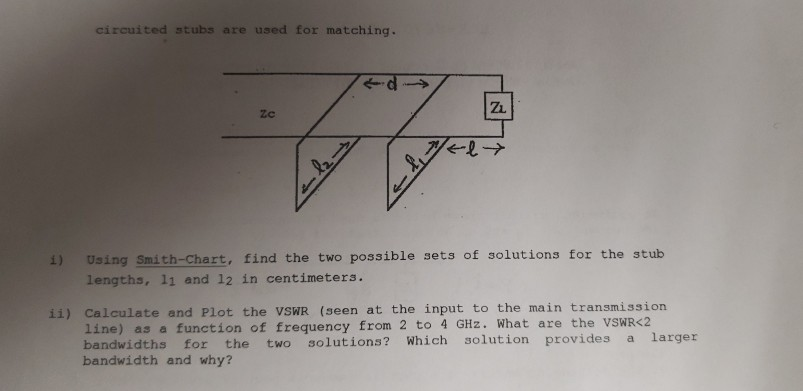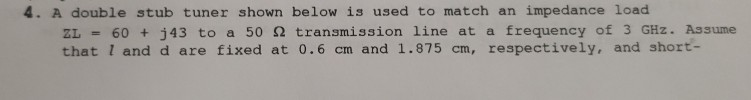# A double stub tuner shown below is used to match an impedance load ZL = 60 + j43 to a 50… 1 answer below »

A double stub tuner shown below is used to match an impedance load ZL = 60 + j43 to a 50 transmission line at a frequency of 3 GHz. Assume that l and d are fixed at 0. 6 cm and 1.875 cm, respectively, and short- %3!

circuited stubs are used for matching. ZL zc 2-> 1) Using Smith-Chart, find the two possible sets of solutions for the stub lengths, l1 and 12 in centimeters. ii) Calculate and Plot the VSWR (seen at the input to the main transmission line) as a function of frequency from 2 to 4 GHz. What are the VSWR1) Using Smith-Chart, find the two possible sets of solutions for the stub l”>